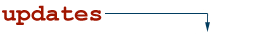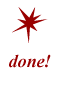# qubit

a.k.a. quantum bit, qubits, quantum bits, qbit, qbits

In quantum computing, a qubit or quantum bit (also seen as qbit) is the basic unit of quantum information. It is the quantum version of the classic binary bit but physically realized as a two-state device.

Examples of a two-state (or two-level) quantum-mechanical system include the spin of the electron in which the two levels can be taken as spin up and spin down, and the polarization of a photon in which the two states can be taken to be the vertical polarization and the horizontal polarization.

In a classic binary system, a bit would have to be in one state or the other. However, quantum mechanics allows the qubit to be in a coherent superposition of both states/levels simultaneously, a property which is fundamental to quantum computing.

NetLingo Classification: Net Technology